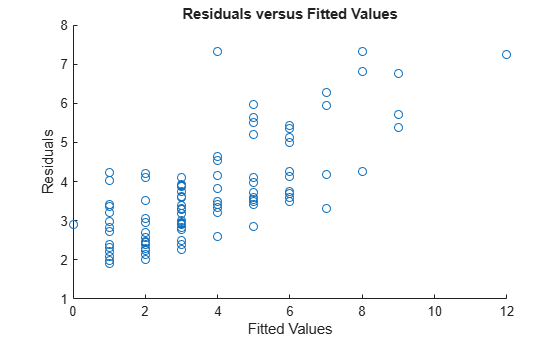# fitted

Fitted responses from generalized linear mixed-effects model

## Syntax

``mufit = fitted(glme)``
``mufit = fitted(glme,Name,Value)``

## Description

example

````mufit = fitted(glme)` returns the fitted conditional response of the generalized linear mixed-effects model `glme`.```
````mufit = fitted(glme,Name,Value)` returns the fitted response with additional options specified by one or more name-value pair arguments. For example, you can specify to compute the marginal fitted response.```

## Input Arguments

expand all

Generalized linear mixed-effects model, specified as a `GeneralizedLinearMixedModel` object. For properties and methods of this object, see `GeneralizedLinearMixedModel`.

### Name-Value Arguments

Specify optional comma-separated pairs of `Name,Value` arguments. `Name` is the argument name and `Value` is the corresponding value. `Name` must appear inside quotes. You can specify several name and value pair arguments in any order as `Name1,Value1,...,NameN,ValueN`.

Indicator for conditional response, specified as the comma-separated pair consisting of `'Conditional'` and one of the following.

ValueDescription
`true`Contributions from both fixed effects and random effects (conditional)
`false`Contribution from only fixed effects (marginal)

To obtain fitted marginal response values, `fitted` computes the conditional mean of the response with the empirical Bayes predictor vector of random effects b set equal to 0. For more information, see Conditional and Marginal Response

Example: `'Conditional',false`

## Output Arguments

expand all

Fitted response values, returned as an n-by-1 vector, where n is the number of observations.

## Examples

expand all

`load mfr`

This simulated data is from a manufacturing company that operates 50 factories across the world, with each factory running a batch process to create a finished product. The company wants to decrease the number of defects in each batch, so it developed a new manufacturing process. To test the effectiveness of the new process, the company selected 20 of its factories at random to participate in an experiment: Ten factories implemented the new process, while the other ten continued to run the old process. In each of the 20 factories, the company ran five batches (for a total of 100 batches) and recorded the following data:

• Flag to indicate whether the batch used the new process (`newprocess`)

• Processing time for each batch, in hours (`time`)

• Temperature of the batch, in degrees Celsius (`temp`)

• Categorical variable indicating the supplier (`A`, `B`, or `C`) of the chemical used in the batch (`supplier`)

• Number of defects in the batch (`defects`)

The data also includes `time_dev` and `temp_dev`, which represent the absolute deviation of time and temperature, respectively, from the process standard of 3 hours at 20 degrees Celsius.

Fit a generalized linear mixed-effects model using `newprocess`, `time_dev`, `temp_dev`, and `supplier` as fixed-effects predictors. Include a random-effects term for intercept grouped by `factory`, to account for quality differences that might exist due to factory-specific variations. The response variable `defects` has a Poisson distribution, and the appropriate link function for this model is log. Use the Laplace fit method to estimate the coefficients. Specify the dummy variable encoding as `'effects'`, so the dummy variable coefficients sum to 0.

The number of defects can be modeled using a Poisson distribution

`${\text{defects}}_{ij}\sim \text{Poisson}\left({\mu }_{ij}\right)$`

This corresponds to the generalized linear mixed-effects model

`$\mathrm{log}\left({\mu }_{ij}\right)={\beta }_{0}+{\beta }_{1}{\text{newprocess}}_{ij}+{\beta }_{2}{\text{time}\text{_}\text{dev}}_{ij}+{\beta }_{3}{\text{temp}\text{_}\text{dev}}_{ij}+{\beta }_{4}{\text{supplier}\text{_}\text{C}}_{ij}+{\beta }_{5}{\text{supplier}\text{_}\text{B}}_{ij}+{b}_{i},$`

where

• ${\text{defects}}_{ij}$ is the number of defects observed in the batch produced by factory $i$ during batch $j$.

• ${\mu }_{ij}$ is the mean number of defects corresponding to factory $i$ (where $i=1,2,...,20$) during batch $j$ (where $j=1,2,...,5$).

• ${\text{newprocess}}_{ij}$, ${\text{time}\text{_}\text{dev}}_{ij}$, and ${\text{temp}\text{_}\text{dev}}_{ij}$ are the measurements for each variable that correspond to factory $i$ during batch $j$. For example, ${\text{newprocess}}_{ij}$ indicates whether the batch produced by factory $i$ during batch $j$ used the new process.

• ${\text{supplier}\text{_}\text{C}}_{ij}$ and ${\text{supplier}\text{_}\text{B}}_{ij}$ are dummy variables that use effects (sum-to-zero) coding to indicate whether company `C` or `B`, respectively, supplied the process chemicals for the batch produced by factory $i$ during batch $j$.

• ${b}_{i}\sim N\left(0,{\sigma }_{b}^{2}\right)$ is a random-effects intercept for each factory $i$ that accounts for factory-specific variation in quality.

```glme = fitglme(mfr,'defects ~ 1 + newprocess + time_dev + temp_dev + supplier + (1|factory)', ... 'Distribution','Poisson','Link','log','FitMethod','Laplace','DummyVarCoding','effects');```

Generate the fitted conditional mean values for the model.

`mufit = fitted(glme);`

Create a scatterplot of the observed values versus fitted values.

```figure scatter(mfr.defects,mufit) title('Residuals versus Fitted Values') xlabel('Fitted Values') ylabel('Residuals')```